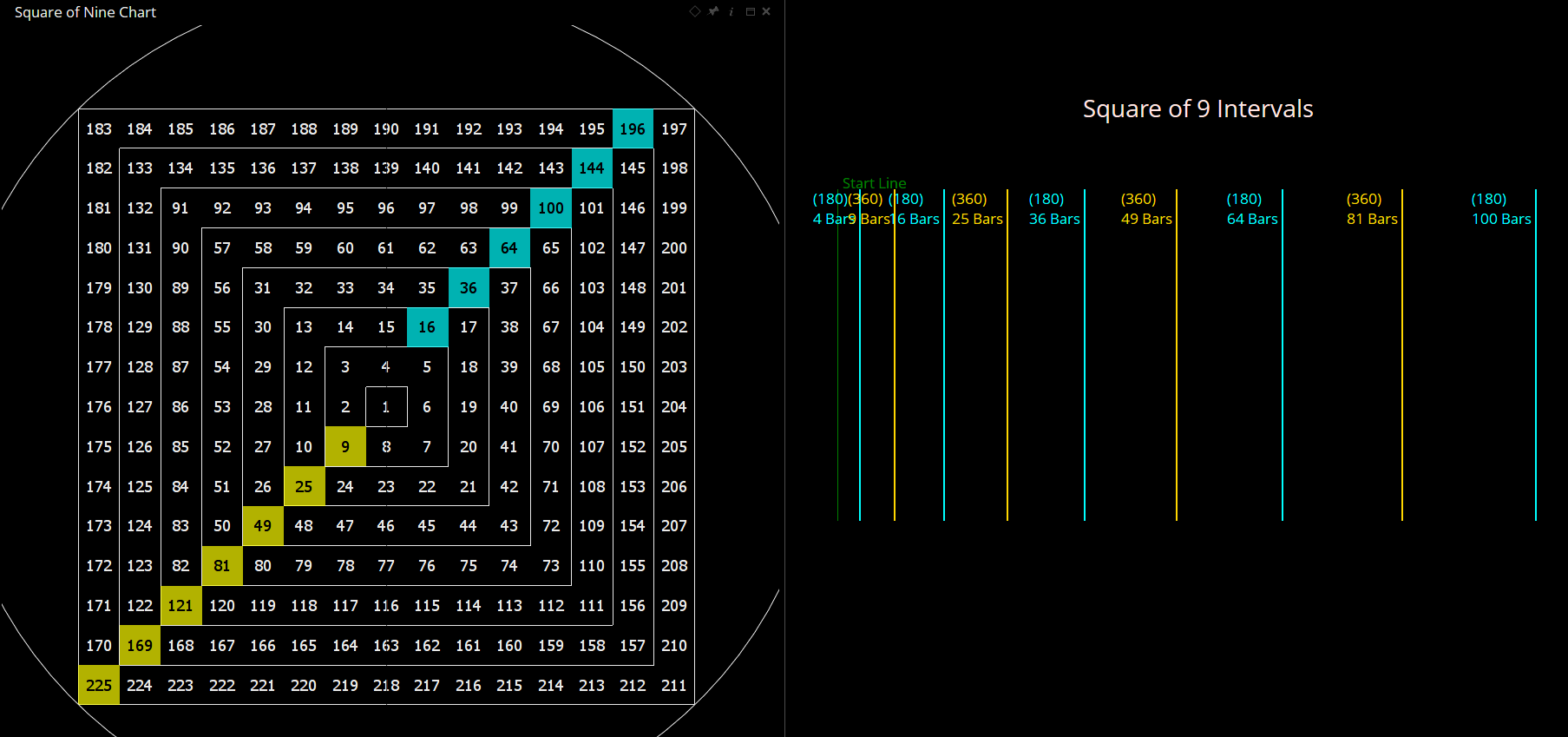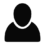Select Page

# Square of Nine

Viewing 3 posts - 1 through 3 (of 3 total)
• Author
Posts
• #63221

Hi,

I have a special question about the tool “Square of 9 Intervals”.

On the “normal” Square of Nine the number sequence: 16, 36, 64, 100, 144, 196, 256, 324, 400, … corresponds to the angel of 135 degree.

When I use the tool “Square of 9 Intervals” I have to select in the properties settings “Square of 9 Angles” the angle 180 degrees to get the above mentioned number sequence. That means in the tool “Square of 9 Intervals” the angle is “shifted forward” 45 degrees. All calculated dates are the same but the angle is different.

That is also the case with all other angles in the tool “Square of 9 Intervals”. They are all “shifted forward” 45 degrees in relation to the original Square of Nine.

Can someone explain me this 45 degree “shift forward” of the angles in the tool “Square of 9 Intervals”?

Thanks,
Thomas

#63251

Hi Thomas,

It’s because of the way the angles are calculated from a starting point of 1.

360° around the wheel is calculated as (√(n)+2)²

so where n=1:

(1+2)² = 9

180° around the wheel is calculated as (√(n)+1

Therefore, if 9 is the 1st 360° level, the tool calculates 180° from there as follows:

(√(9)+1)² = 16

The next 360° value around the square from 9 is calculated as (3+2)² = 25.

Keep repeating the calculations and you get the squares of the odd numbers (9, 25, 49, 81, etc) expanding to the bottom left, with the even numbers (16, 36, 64, 100, etc) appearing opposite ie 180° heading top-right.

I hope that makes sense.###### 1 user thanked author for this post.Thomas
#63253

Thanks a million Darren,

Thomas

Viewing 3 posts - 1 through 3 (of 3 total)
• You must be logged in to reply to this topic.